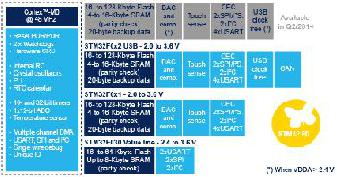STM32F0 Standard Peripheral bibliotheek  1.0 ST Microelectronics standard peripheral bibliotheek documentatie voor de STM32F0 familie
stm32f0xx_gpio.h File Reference

This file contains all the functions prototypes for the GPIO firmware library. More...

`#include "stm32f0xx.h"`

Go to the source code of this file.

## Data Structures

struct  GPIO_InitTypeDef
GPIO Init structure definition. More...

## Macros

#define IS_GPIO_ALL_PERIPH(PERIPH)

#define IS_GPIO_LIST_PERIPH(PERIPH)

#define IS_GPIO_MODE(MODE)

#define IS_GPIO_OTYPE(OTYPE)   (((OTYPE) == GPIO_OType_PP) || ((OTYPE) == GPIO_OType_OD))

#define IS_GPIO_SPEED(SPEED)

#define IS_GPIO_PUPD(PUPD)

#define IS_GPIO_BIT_ACTION(ACTION)   (((ACTION) == Bit_RESET) || ((ACTION) == Bit_SET))

#define GPIO_Pin_0   ((uint16_t)0x0001)

#define GPIO_Pin_1   ((uint16_t)0x0002)

#define GPIO_Pin_2   ((uint16_t)0x0004)

#define GPIO_Pin_3   ((uint16_t)0x0008)

#define GPIO_Pin_4   ((uint16_t)0x0010)

#define GPIO_Pin_5   ((uint16_t)0x0020)

#define GPIO_Pin_6   ((uint16_t)0x0040)

#define GPIO_Pin_7   ((uint16_t)0x0080)

#define GPIO_Pin_8   ((uint16_t)0x0100)

#define GPIO_Pin_9   ((uint16_t)0x0200)

#define GPIO_Pin_10   ((uint16_t)0x0400)

#define GPIO_Pin_11   ((uint16_t)0x0800)

#define GPIO_Pin_12   ((uint16_t)0x1000)

#define GPIO_Pin_13   ((uint16_t)0x2000)

#define GPIO_Pin_14   ((uint16_t)0x4000)

#define GPIO_Pin_15   ((uint16_t)0x8000)

#define GPIO_Pin_All   ((uint16_t)0xFFFF)

#define IS_GPIO_PIN(PIN)   ((PIN) != (uint16_t)0x00)

#define IS_GET_GPIO_PIN(PIN)

#define GPIO_PinSource0   ((uint8_t)0x00)

#define GPIO_PinSource1   ((uint8_t)0x01)

#define GPIO_PinSource2   ((uint8_t)0x02)

#define GPIO_PinSource3   ((uint8_t)0x03)

#define GPIO_PinSource4   ((uint8_t)0x04)

#define GPIO_PinSource5   ((uint8_t)0x05)

#define GPIO_PinSource6   ((uint8_t)0x06)

#define GPIO_PinSource7   ((uint8_t)0x07)

#define GPIO_PinSource8   ((uint8_t)0x08)

#define GPIO_PinSource9   ((uint8_t)0x09)

#define GPIO_PinSource10   ((uint8_t)0x0A)

#define GPIO_PinSource11   ((uint8_t)0x0B)

#define GPIO_PinSource12   ((uint8_t)0x0C)

#define GPIO_PinSource13   ((uint8_t)0x0D)

#define GPIO_PinSource14   ((uint8_t)0x0E)

#define GPIO_PinSource15   ((uint8_t)0x0F)

#define IS_GPIO_PIN_SOURCE(PINSOURCE)

#define GPIO_AF_0
AF 0 selection. More...

#define GPIO_AF_1
AF 1 selection. More...

#define GPIO_AF_2   ((uint8_t)0x02) /* TIM2, TIM1, EVENTOUT, TIM16, TIM17 */
AF 2 selection.

#define GPIO_AF_3   ((uint8_t)0x03) /* TS, I2C1, TIM15, EVENTOUT */
AF 3 selection.

#define GPIO_AF_4   ((uint8_t)0x04) /* TIM14 */
AF 4 selection.

#define GPIO_AF_5   ((uint8_t)0x05) /* TIM16, TIM17 */
AF 5 selection.

#define GPIO_AF_6   ((uint8_t)0x06) /* EVENTOUT */
AF 6 selection.

#define GPIO_AF_7   ((uint8_t)0x07) /* COMP1 OUT and COMP2 OUT */
AF 7 selection.

#define IS_GPIO_AF(AF)

#define GPIO_Speed_10MHz   GPIO_Speed_Level_1

#define GPIO_Speed_2MHz   GPIO_Speed_Level_2

#define GPIO_Speed_50MHz   GPIO_Speed_Level_3

## Enumerations

enum  GPIOMode_TypeDef { GPIO_Mode_IN = 0x00, GPIO_Mode_OUT = 0x01, GPIO_Mode_AF = 0x02, GPIO_Mode_AN = 0x03 }

enum  GPIOOType_TypeDef { GPIO_OType_PP = 0x00, GPIO_OType_OD = 0x01 }

enum  GPIOSpeed_TypeDef { GPIO_Speed_Level_1 = 0x01, GPIO_Speed_Level_2 = 0x02, GPIO_Speed_Level_3 = 0x03 }

enum  GPIOPuPd_TypeDef { GPIO_PuPd_NOPULL = 0x00, GPIO_PuPd_UP = 0x01, GPIO_PuPd_DOWN = 0x02 }

enum  BitAction { Bit_RESET = 0, Bit_SET }

## Functions

void GPIO_DeInit (GPIO_TypeDef *GPIOx)
Deinitializes the GPIOx peripheral registers to their default reset values. More...

void GPIO_Init (GPIO_TypeDef *GPIOx, GPIO_InitTypeDef *GPIO_InitStruct)
Initializes the GPIOx peripheral according to the specified parameters in the GPIO_InitStruct. More...

void GPIO_StructInit (GPIO_InitTypeDef *GPIO_InitStruct)
Fills each GPIO_InitStruct member with its default value. More...

void GPIO_PinLockConfig (GPIO_TypeDef *GPIOx, uint16_t GPIO_Pin)
Locks GPIO Pins configuration registers. More...

uint8_t GPIO_ReadInputDataBit (GPIO_TypeDef *GPIOx, uint16_t GPIO_Pin)
Reads the specified input port pin. More...

uint16_t GPIO_ReadInputData (GPIO_TypeDef *GPIOx)
Reads the specified input port pin. More...

uint8_t GPIO_ReadOutputDataBit (GPIO_TypeDef *GPIOx, uint16_t GPIO_Pin)
Reads the specified output data port bit. More...

uint16_t GPIO_ReadOutputData (GPIO_TypeDef *GPIOx)
Reads the specified GPIO output data port. More...

void GPIO_SetBits (GPIO_TypeDef *GPIOx, uint16_t GPIO_Pin)
Sets the selected data port bits. More...

void GPIO_ResetBits (GPIO_TypeDef *GPIOx, uint16_t GPIO_Pin)
Clears the selected data port bits. More...

void GPIO_WriteBit (GPIO_TypeDef *GPIOx, uint16_t GPIO_Pin, BitAction BitVal)
Sets or clears the selected data port bit. More...

void GPIO_Write (GPIO_TypeDef *GPIOx, uint16_t PortVal)
Writes data to the specified GPIO data port. More...

void GPIO_PinAFConfig (GPIO_TypeDef *GPIOx, uint16_t GPIO_PinSource, uint8_t GPIO_AF)
Writes data to the specified GPIO data port. More...

## Detailed Description

This file contains all the functions prototypes for the GPIO firmware library.

Version
V1.0.1
Date
20-April-2012
Attention

```   http://www.st.com/software_license_agreement_liberty_v2